蚁群算法---matlab代码

5 篇文章 0 订阅

摘来理论部分（我比较懒，图片是摘自司守奎的《数学建模算法与应用》）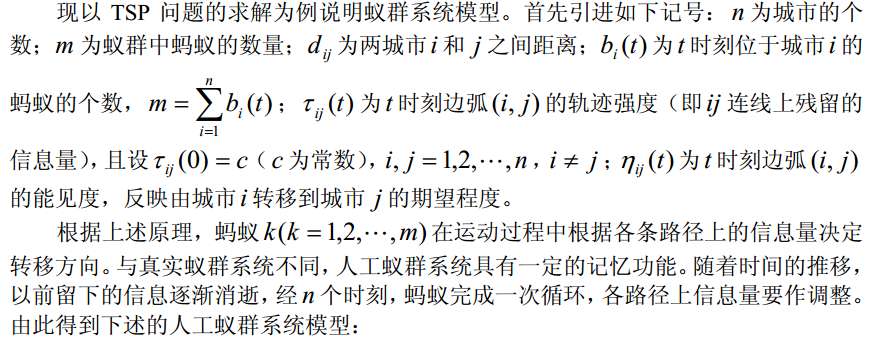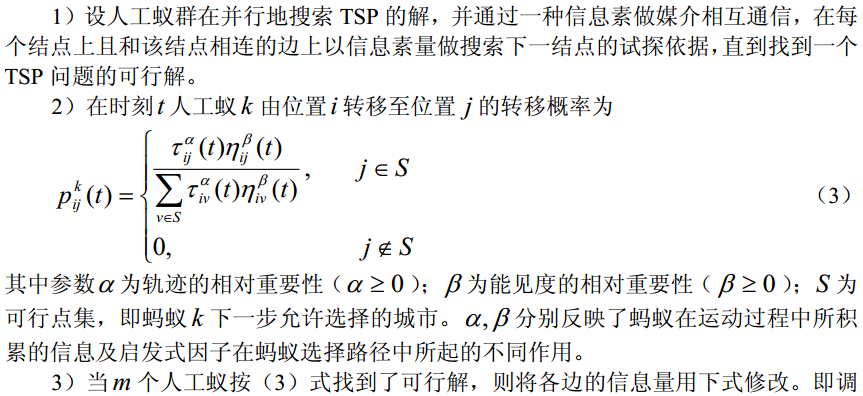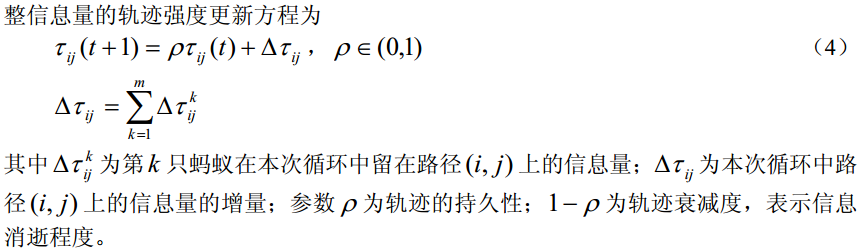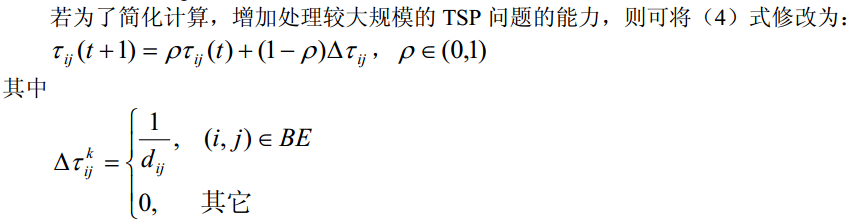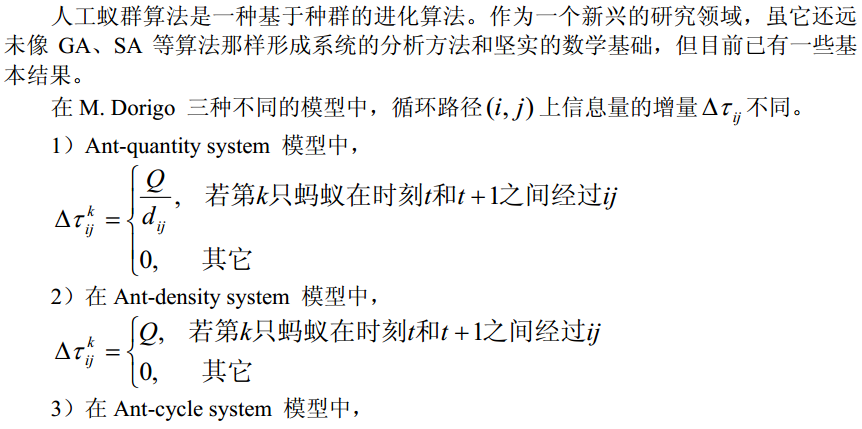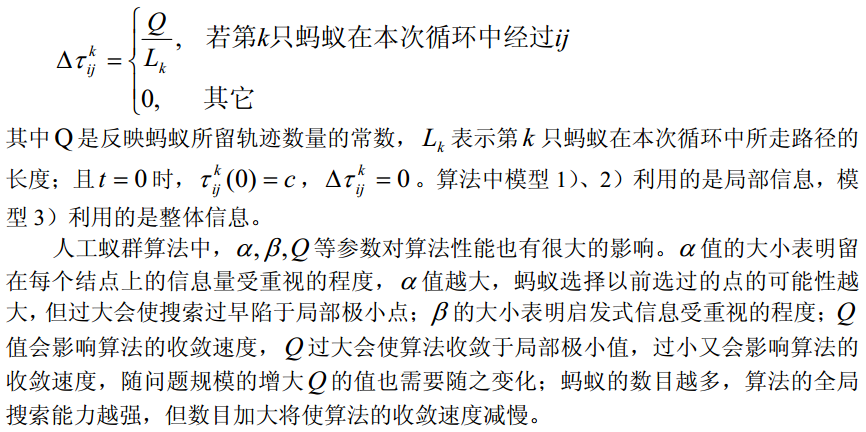代码说明

在网上无意间找到了这个代码，写的非常不错：依照原理论一板一眼的写的代码，没有偷工减料，很难得。但是看起来比较费劲，就自己改了下：

• 删改了原代码批注，并增加了每一行代码的注释，相信再接触这份代码理解起来会快一些
• 精简了两三处代码
• 改了下变量名称，总觉得原来的看着乱

Matlab代码

City = rand(20,2).*randi([1,100],20,2);
Ite = 200;
Ant_num = 40;
Alpha = 0.7;
Beta = 0.9;
Rho = 0.3;
Q = 100;
[R_best,L_best,L_ave,Shortest_Route,Shortest_Length]=ACATSP(City,Ite,Ant_num,Alpha,Beta,Rho,Q)

function [R_best,L_best,L_ave,Shortest_Route,Shortest_Length]=ACATSP(City,Ite,Ant_num,Alpha,Beta,Rho,Q)
%% 主要符号说明
% City n个城市的坐标，n×2的矩阵
% Ite 最大迭代次数
% Ant_num 蚂蚁个数
% Alpha 表征信息素重要程度的参数
% Beta 表征启发式因子重要程度的参数
% Eta 启发因子，这里设为距离的倒数
% Tau 信息素矩阵
% Rho 信息素蒸发系数
% Q 信息素增加强度系数
% R_best 各代最佳路线
% L_best 各代最佳路线的长度

%% 第一步：变量初始化
City_num = size(City,1);%n表示问题的规模（城市个数）
Distance = zeros(City_num,City_num);%D表示完全图的赋权邻接矩阵

for i = 1:City_num
for j = 1:City_num
if i ~= j
Distance(i,j) = sqrt( (City(i,1)-City(j,1))^2 + (City(i,2)-City(j,2))^2 );% 计算任意两点间距离
else
Distance(i,j) = eps;      % i=j时不计算，应该为0，但后面的启发因子要取倒数，用eps（浮点相对精度）表示
end
Distance(j,i) = Distance(i,j);   % 对称矩阵
end
end

Eta = 1./Distance;          % Eta为启发因子，这里设为距离的倒数
Tau = ones(City_num,City_num);     % Tau为信息素矩阵
Ite_num = 1;               % 迭代计数器，记录迭代次数
R_rec = zeros(Ant_num,City_num);   % 存储并记录路径的生成
R_best = zeros(Ite,City_num);       % 各代最佳路线
L_best = inf.*ones(Ite,1);   % 各代最佳路线的长度
L_ave = zeros(Ite,1);        % 各代路线的平均长度

while Ite_num<=Ite        % 停止条件之一：达到最大迭代次数，停止

%% 第二步：将Ant_num只蚂蚁放到City_num个城市上
Init_ant_position = [];   % 将初始状态下的蚂蚁随机分配到各个城市的临时矩阵。
for i = 1:( ceil(Ant_num/City_num) )
Init_ant_position = [Init_ant_position,randperm(City_num)];
% 每次迭代将蚂蚁随机分配到所有城市。生成一个1行多列（由于ceiling向上取整，则列数大于等于蚂蚁个数）的矩阵。
end
R_rec(:,1) = (Init_ant_position(1,1:Ant_num))';
% 矩阵转置后变成 Ant_num行-1列的一个一个初始矩阵，每一行代表一只蚂蚁，每个值代表当前蚂蚁所在城市代码。

%% 第三步：Ant_num只蚂蚁按概率函数选择下一座城市，完成各自的周游
% 这里说明下：这是个两重的大循环，外层是城市，内层是蚂蚁。
% 也就是说每次迭代每只蚂蚁向前走一步，而不是一只蚂蚁走完所有城市再开始下一只。
for j = 2:City_num     % 所在城市不计算
for i = 1:Ant_num    % 对每一只蚂蚁
City_visited = R_rec(i,1:(j-1));   % 记录已访问的城市，避免重复访问
City_unvisited = zeros(1,(City_num-j+1));  % 待访问的城市，初始为空
P = City_unvisited;   % 待访问城市的选择概率分布，我猜这里作者弄了个简便写法，其实只是想弄一个同型矩阵。
count = 1; % 待访问城市 City_unvisited 的下标计数器

% 统计未去过的城市
for k = 1:City_num
if isempty( find( City_visited == k, 1 ) )
% 如果去过k城市，则find不为空,find(x,1)的意思是找到第一个就结束，是一个提高运算性能的写法。
% 这句话是为了避免重复去同一个城市。
City_unvisited(count) = k; % 如果if判断为真，说明第k 个城市没有去过。
count = count+1;      % 下标计数器加1
end
end

% 下面计算待选城市的概率分布
for k = 1:length(City_unvisited)
P(k) = ( Tau( City_visited(end), City_unvisited(k) )^Alpha )*...
( Eta( City_visited(end), City_unvisited(k) )^Beta );
end
P=P/(sum(P));

% 按概率原则选取下一个城市
P_cum = cumsum(P);  % cumsum函数是一个比较特殊的求和函数，这里是得到P 的累积概率矩阵。
Select = find(P_cum>=rand); % 若计算的概率大于原来的就选择这条路线
To_visit = City_unvisited(Select(1)); % Select(1)的意思是选中第一个累积概率大于rand随机数的城市
R_rec(i,j) = To_visit;  % R_rec(i,j) 是指第i只蚂蚁，第将要去j步将要去的那个城市，循环结束后得到每只蚂蚁的路径
end
end

% 如果不是第一次循环，则将最优路径赋给路径记录矩阵的第一行
if Ite_num >= 2
R_rec(1,:) = R_best(Ite_num-1,:);
end

%% 第四步：记录本次迭代最佳路线
Len = zeros(Ant_num,1);     % length 距离矩阵，初始为0。记录每只蚂蚁当前路径的总距离。

for i=1:Ant_num
R_temp = R_rec(i,:); % 取得第i 只蚂蚁的路径
% 计算第i只蚂蚁走过的总距离
for j = 1:(City_num-1)
Len(i) = Len(i) + Distance( R_temp(j),R_temp(j+1) );  % 原距离加上第j个城市到第j+1个城市的距离
end
Len(i)=Len(i)+Distance(R_temp(1),R_temp(City_num));      % 一轮下来后走过的距离
end

[L_best(Ite_num), index] = min(Len);     % 最佳距离取最小
R_best(Ite_num,:) = R_rec(index(1), :);
% 此轮迭代后的最佳路线。为什么是index(1)，这是严谨写法：因为min求出后如果有多个最小值则index不唯一。
L_ave(Ite_num) = mean(Len);           % 此轮迭代后的平均距离
Ite_num=Ite_num+1;                      % 迭代继续

%% 第五步：更新信息素
Delta_Tau = zeros(City_num, City_num);        % 开始时信息素为n*n的0矩阵

for i = 1:Ant_num
for j = 1:(City_num-1)
Delta_Tau(R_rec(i,j), R_rec(i,j+1)) = Delta_Tau(R_rec(i,j), R_rec(i,j+1))+Q/Len(i);
%此次循环在路径（i，j）上的信息素增量
end
Delta_Tau(R_rec(i,City_num), R_rec(i,1)) = Delta_Tau(R_rec(i,City_num), R_rec(i,1))+Q/Len(i);
%此次循环在整个路径上的信息素增量
end

Tau = (1-Rho).*Tau + Delta_Tau; %考虑信息素挥发，更新后的信息素  Rho 信息素蒸发系数

%% 第六步：禁忌表清零
R_rec=zeros(Ant_num,City_num);             %%直到最大迭代次数

end

%% 第七步：输出结果

Pos = find(L_best==min(L_best)); % 找到最佳路径（非0为真）
Shortest_Route=R_best(Pos(1), :) % 最大迭代次数后最佳路径
Shortest_Length=L_best(Pos(1) ) % 最大迭代次数后最短距离

figure                % 绘制第一个子图形
DrawRoute(City,Shortest_Route)     % 画路线图的子函数

figure                % 绘制第二个子图形
plot(L_best)
hold on                         % 保持图形
plot(L_ave,'r')
title('平均距离和最短距离')     % 标题

function DrawRoute(C,R)
%% 画路线图的子函数
% C Coordinate 节点坐标，由一个N×2的矩阵存储
% R Route 路线

N=length(R);
scatter(C(:,1),C(:,2));
hold on
plot([C(R(1),1),C(R(N),1)],[C(R(1),2),C(R(N),2)],'g')

for ii=2:N
plot([C(R(ii-1),1),C(R(ii),1)],[C(R(ii-1),2),C(R(ii),2)],'g')
end
title('旅行商问题优化结果 ')
hold off
02-0711-052万+
05-02
11-266444
04-273万+
03-061万+
04-20
03-212050
07-096155
09-087万+
05-095万+
08-31923点击重新获取扫码支付余额充值